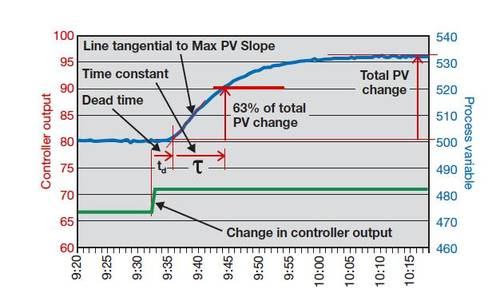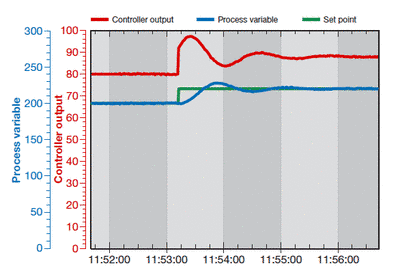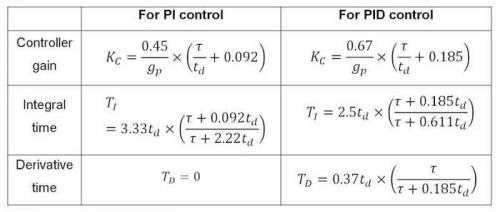# Industry News Display

2014-07-01   Control Engineering

## When choosing a tuning strategy for a specific control loop, it is important to match the technique to the needs of that loop and the larger process. It is also important to have more than one approach in your repertoire, and the Cohen-Coon method can be a handy addition in the right situation.

By: Lee Payne

The well-known Ziegler-Nichols tuning rules work well only on processes with very long time constants relative to their dead times, and on level control loops. However, its performance is not good on flow, liquid pressure, and many other loops that require fast adjustment. In contrast, the Cohen-Coon tuning rules work well on virtually all self-regulating processes and were designed to give a very fast response.

The method's original design resulted in loops with too much oscillatory response and consequently fell into disuse. However, with some modification, Cohen-Coon tuning rules proved their value for control loops that need to respond quickly while being much less prone to oscillations.

Applicable process types

The Cohen-Coon tuning method isn't suitable for every application. For starters, it can be used only on self-regulating processes. Most control loops, e.g., flow, temperature, pressure, speed, and composition, are, at least to some extent, self-regulating processes. (On the other hand, the most common integrating process is a level control loop.)A self-regulating process always stabilizes at some point of equilibrium, which depends on the process design and the controller output. If the controller output is set to a different value, the process will respond and stabilize at a new point of equilibrium.

Target controller algorithm

Cohen-Coon tuning rules have been designed for use on a non-interactive controller algorithm such as that provided by the Dataforth MAQ 20 industrial data acquisition and control system. There are controllers with similar characteristics available from other suppliers.

Procedure

To apply modified Cohen-Coon tuning rules, follow the steps below. The process variable and controller output must be time-trended so that measurements can be taken from them.

1. Do a controller output step test:

• Put the controller in manual and wait for the process to settle out.
• Make a step change in the CO (controller output) of a few percent and wait for the PV (process variable) to settle out. The size of this step should be large enough that the PV moves well clear of the process noise and disturbance level. A total movement of five times more than the peak-to-peak level of the noise and disturbances on the PV should be sufficient.2. Determine the process characteristics (see Figure 1):

• If the PV is not ranged 0-100%, convert the change in PV to a percentage of the range: change in PV [in %] = change in PV [in engineering units] × 100 / (PV upper calibration limit - PV lower calibration limit).
• Calculate the process gain (gp): gp = total change in PV [in %] / change in CO [in %].
• Find the maximum slope of the PV response curve. This will be at the point of inflection. Draw a tangential line through the PV response curve at this point.
• Extend this line to intersect with the original level of the PV before the step in CO.
• Take note of the time value at this intersection and calculate the dead time (td): td = time difference between the change in CO and the intersection of the tangential line and the original PV level.
• If td was measured in seconds, divide it by 60 to convert it to minutes. (Since the Dataforth PID controller uses minutes as its time base for integral time, all measurements have to be made in minutes or converted to minutes. Many other controllers are similar.)
• Calculate the value of the PV at 63% of its total change.
• On the PV reaction curve, find the time value at which the PV reaches this level.
• Calculate the time constant (t): t = time difference between intersection at the end of dead time and the PV reaching 63% of its total change.
• If t was measured in seconds, divide it by 60 to convert it to minutes.

3. Repeat steps 1 and 2 three more times to obtain good average values for the process characteristics.

4. Calculate controller settings for a PI or PID controller using the modified Cohen-Coon equations below. (The modified rules calculate the controller gain as ½ of that calculated by the original rules.)5. Enter the values into the controller, make sure the algorithm is set to non-interactive, and put the controller in automatic mode.

6. Change the setpoint to test the new values.

Do fine tuning if necessary. The control loop's response can be slowed down and made less oscillatory, if needed, by decreasing KC and/or increasing TI.

Conclusion

These modified Cohen-Coon tuning rules are an excellent method for achieving fast response on virtually all control loops with self-regulating processes. They are an effective and highly reliable alternative to the Ziegler-Nichols tuning method, which does not work well when applied to many self-regulating processes.

Lee Payne is CEO of Dataforth.

Additional reading: G.H. Cohen and G.A. Coon, Theoretical Consideration of Retarded Control, Trans. ASME, 75, pp. 827-834, 1953.

ONLINE